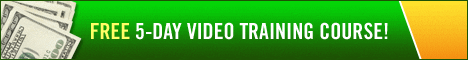# Forecasting the Future Value of Your IRA316

Curious about how much money you’ll accumulate in your individual retirement account (IRA)? Just use Microsoft Excel to calculate a pretty good estimate says author and CPA Stephen L. Nelson.

IRA, individual retirement account, retirement, investing

If you’ve got Microsoft Excel (or just about any other popular spreadsheet program) running on your computer, you can use its FV function to forecast the future value of your IRA account.

The FV function calculates the future value of an investment given its interest rate,
the number of payments, the payment, the present value of the investment, and,
optionally, the type-of-annuity switch. (More about the type-of-annuity switch a little later.)

The function uses the following syntax:

=FV(rate,nper,pmt,pv,type)

This little pretty complicated, I grant you. But suppose you want to calculate the future value of an IRA account that’s already got \$10,000 in it and to which you’re contributing \$200-a-month. Further suppose that you want to know the account balance-its future value-in 25 years and that you expect to earn 10% annual interest.

To calculate the future value of the IRA account in this case using the FV function, you enter the following into a worksheet cell:

=FV(10%/12,25*12,-200,-10000,0)

The function returns the value 385936.13-roughly \$386,000 dollars.

A handful of things to note: To convert the 10% annual interest to a monthly interest rate, the formula divides the annual interest rate by 12. Similarly, to convert the 25-year term to a term in months, the formula multiplies 25 by 12.

Also, notice that the monthly payment and initial present values show as negative amounts because they represent cash outflows. And the function returns the future value amount as a positive value because it reflects a cash inflow the investor ultimately receives.

That 0 at the end of the function is the type-of-annuity switch. If you set the type-of-annuity switch to 1, Excel assumes payments occur at the beginning of the period (month in this case), following the annuity due convention. If you set the annuity switch to 0 or you omit the argument, Excel assumes payments occur at the end of the period following the ordinary annuity convention.